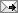## Wednesday, January 9, 2008

Elasticities in Regressions. (update of old post)Here are how to calculate elasticities from regression coefficients, a note possibly useful to economists who like me keep having to rederive this basic method:
1. The elasticity is (%change in Y)/(%change in X) = (dy/dx)*(x/y).
2. If y = beta*x then the elasticity is beta*(x/y).
3. If y = beta* log(x) then the elasticity is (beta/x)*(x/y) = beta/y.
4. If log(y) = beta* log(x) then the elasticity is (beta*y/x)*(x/y) = beta, which is a constant elasticity.
(reason: then y= exp(beta*log(x)), so dy/dx = beta*exp(beta*log(x))*(1/x) = beta*y/x.)
5. If log(y) = beta*x then the elasticity is (beta* y )*(x/y) = beta*x.
(reason: then y = exp(beta*x), so dy/dx = beta*exp(beta*x) = beta*y.)

6. If log(y) = alpha + beta*D, where D is a dummy variable, then we are interested in the finite jump from D=0 to D=1, not an infinitesimal elasticity. That percentage jump is

dy/y = exponent(beta)-1,

because log(y,D=0) = alpha and log(y, D=1) = alpha + beta, so

(y,D=1)/(y, D=0) = exp(alpha+beta)/exp(alpha) = exp(beta)

and

dy/y = (y,D=1)/(y, D=0) -1 = exp(beta)-1

This is consistent, but not unbiased. We know that OLS is BLUE, unbiased, as an estimator of the impact of the dummy D on log(Y), but that does not imply that it is unbiased as an estimator of the impact of D on Y. That is because E(f(z)) does not equal f(E(z)) in general and that ultimate effect of D on y, exp(beta)-1, is a nonlinear function of beta. Alexander Borisov pointed out to me that Peter Kennedy (AER, 1981) suggests using exp(betahat-vhat(betahat)/2)-1 as an estimate of the effect of going from D=0 to D=1, as biased, but less biased, and also consistent .

Labels: ,

To view the post on a separate page, click: at (the permalink).